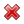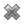# Fight Finance

#### CoursesTagsRandomAllRecentScoresScoreskeithphw $6,011.61 Jade$1,815.80 Chu $789.98 royal ne...$750.00 Leehy $713.33 Visitor$650.00 ZOE HY $640.00 JennyLI$625.61 Visitor $590.00 Visitor$555.33 Visitor $550.00 Visitor$550.00 Visitor $540.00 Visitor$500.00 Yizhou $489.18 Visitor$480.00 Visitor $470.00 Visitor$464.70 Visitor $460.00 Jasper.sun$460.00

A stock is expected to pay the following dividends:

 Cash Flows of a Stock Time (yrs) 0 1 2 3 4 ... Dividend ($) 0.00 1.15 1.10 1.05 1.00 ... After year 4, the annual dividend will grow in perpetuity at -5% pa. Note that this is a negative growth rate, so the dividend will actually shrink. So, • the dividend at t=5 will be $1(1-0.05) = 0.95$, • the dividend at t=6 will be $1(1-0.05)^2 = 0.9025$, and so on. The required return on the stock is 10% pa. Both the growth rate and required return are given as effective annual rates. What will be the price of the stock in four and a half years (t = 4.5)? A firm can issue 5 year annual coupon bonds at a yield of 8% pa and a coupon rate of 12% pa. The beta of its levered equity is 1. Five year government bonds yield 5% pa with a coupon rate of 6% pa. The market's expected dividend return is 4% pa and its expected capital return is 6% pa. The firm's debt-to-equity ratio is 2:1. The corporate tax rate is 30%. What is the firm's after-tax WACC? Assume a classical tax system. An 'interest payment' is the same thing as a 'coupon payment'. or ? Examine the following graph which shows stocks' betas $(\beta)$ and expected returns $(\mu)$:Assume that the CAPM holds and that future expectations of stocks' returns and betas are correctly measured. Which statement is NOT correct? In these tough economic times, central banks around the world have cut interest rates so low that they are practically zero. In some countries, government bond yields are also very close to zero. A three year government bond with a face value of$100 and a coupon rate of 2% pa paid semi-annually was just issued at a yield of 0%. What is the price of the bond?

A pharmaceutical firm has just discovered a valuable new drug. So far the news has been kept a secret.

The net present value of making and commercialising the drug is $200 million, but$600 million of bonds will need to be issued to fund the project and buy the necessary plant and equipment.

The firm will release the news of the discovery and bond raising to shareholders simultaneously in the same announcement. The bonds will be issued shortly after.

Once the announcement is made and the bonds are issued, what is the expected increase in the value of the firm's assets (ΔV), market capitalisation of debt (ΔD) and market cap of equity (ΔE)?

The triangle symbol is the Greek letter capital delta which means change or increase in mathematics.

Ignore the benefit of interest tax shields from having more debt.

Remember: $ΔV = ΔD+ΔE$

A 4.5% fixed coupon Australian Government bond was issued at par in mid-April 2009. Coupons are paid semi-annually in arrears in mid-April and mid-October each year. The face value is \$1,000. The bond will mature in mid-April 2020, so the bond had an original tenor of 11 years.

Today is mid-September 2015 and similar bonds now yield 1.9% pa.

What is the bond's new price? Note: there are 10 semi-annual coupon payments remaining from now (mid-September 2015) until maturity (mid-April 2020); both yields are given as APR's compounding semi-annually; assume that the yield curve was flat before the change in yields, and remained flat afterwards as well.

Which of the following terms about options are NOT synonyms?

A stock's returns are normally distributed with a mean of 10% pa and a standard deviation of 20 percentage points pa. What is the 90% confidence interval of returns over the next year? Note that the Z-statistic corresponding to a one-tail:

• 90% normal probability density function is 1.282.
• 95% normal probability density function is 1.645.
• 97.5% normal probability density function is 1.960.

The 90% confidence interval of annual returns is between:

A common phrase heard in financial markets is that ‘high risk investments deserve high returns’. To make this statement consistent with the Capital Asset Pricing Model (CAPM), a high amount of what specific type of risk deserves a high return?

Investors deserve high returns when they buy assets with high: